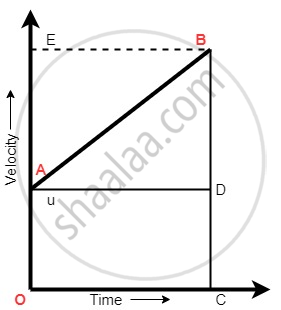Share

# Equations of Motion by Graphical Method - Equation for Position-time Relation

#### notes

Equation of position – Time Relation (2nd equation of motion)Area under slope = Displacement = area of OABC

Area of OABC = Area of rectangle AOCD + Area of ADB

"S" = "OC" xx "OA" + 1/2 xx "AD" xx "BD"

"S" = "OC" xx "OA" + 1/2 "AD" xx("BC – CD")

"S" = "t" xx "u" + 1/2"t" ("v – u") "BC – CD" =" BD"

"S" = "ut" + 1/2"t"("at") "v" ="u + at"

"S" = "ut" + 1/2"at"^2

S